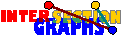# Cliques

As mentioned in Subsection 4.5, line graphs have few cliques. Although not necessary for intersection classes, this property is not unfamiliar. But let us for the moment forget about intersection graphs and treat the following problem: Given is a class G of (finite) graphs. How can we decide whether there is some polnomial f(n) such that every n-vertex graph of the class has at most f(n) cliques? This is a nontrivial property, since the class of all finite graphs does not allow such a polynomial. This can be seen by the so-called octahedron graphs Ot, which are obtained from complete graphs with an even number 2t of vertices by deleting a perfect matching. Every way of picking one vertex of every pair of nonadjacent vertices of Ot gives a clique, thus Ot has 2t vertices but 2t cliques. It turns out that for classes which are closed under induced subgraphs, these graphs are essentially the only obstructions.[BY89], [P95]: Let the class G of (finite) graphs be closed under induced subgraphs.Then there is some polynomial f(n) such that every n-vertex member of G has at most f(n) cliques if and only if there is some t such that Ot} is not in G.

Moreover, we can choose f(n) = n2t-2 in that case.

Listing all c cliques of a graph can be done in time O(nmc) for every graph, thus if Ot is forbidden in our class, we can list all cliques in time O(n2t+1). If there are only few cliques, a maximum clique, or a maximum weighted clique can also be found in the same time. All we have to do is list all cliques and check.

## Cliques in intersection graphs

Since intersection graphs of members of H, where H is closed under subhypergraphs, are closed under induced subgraphs, this result immediately applies to them. Let us just list some examples:

• O2 = C4 is no interval graph,
• Od+1 is no intersection graph of boxes in Rd,
• O4 is no line graph,
There is some topological reason for these facts.

Note that the question of the movie theatre example can now be answered efficiently.

What happens if the number of cliques is exponential? There are intersection classes where the MAXCLIQUE problem is NP-complete, for instance intersection graphs of convex sets in the plane [KK97]. For some classes, as intersection graphs of straight lines, the complexity is open. Sometimes maximum cliques can still be computed efficiently. Examples are circular-arc graphs, but also unit-disk graphs, see here for how to do it.

## Independent sets in intersection graphs

When are complements of large octahedrons, i.e. graphs nK2 forbidden induced subgraphs? This is more seldom, but occurs for instance in intersection graphs of subsets of some fixed finite set. Thus independence number (and also clique number) of graphs of bounded intersection number can be computed in polynomial time.

Back to the start page for intersection graphs.

Erich Prisner